Aggregate soil properties along user-defined slabs, and optionally within groups.

# S4 method for SoilProfileCollection
slab(
object,
fm,
slab.structure = 1,
strict = FALSE,
byhz = TRUE,
slab.fun = slab_function(method = "numeric"),
cpm = 1,
weights = NULL,
...
)

slab_function(
method = c("numeric", "factor", "hd", "weighted.numeric", "weighted.factor", "fast")
)

## Arguments

object

a SoilProfileCollection

fm

A formula: either groups ~ var1 + var2 + var3 where named variables are aggregated within groups' OR where named variables are aggregated across the entire collection  ~ var1 + var2 + var3. If groupsis a factor it must not containNA

slab.structure

A user-defined slab thickness (defined by an integer), or user-defined structure (numeric vector). See details below.

strict

logical: should horizons be strictly checked for self-consistency?

byhz

logical: should horizons or whole profiles be removed by logic checks in strict? Default TRUE removes only offending horizons, FALSE removes whole profiles with one or more illogical horizons.

slab.fun

Function used to process each 'slab' of data, ideally returning a vector with names attribute. Defaults to a wrapper function around stats::quantile(). See details.

cpm

Strategy for normalizing slice-wise probabilities, dividing by either: number of profiles with data at the current slice (cpm=1), or by the number of profiles in the collection (cpm=2). Mode 1 values will always sum to the contributing fraction, while mode 2 values will always sum to 1.

weights

Column name containing site-level weights

...

further arguments passed to slab.fun

method

one of "numeric", "factor", "hd", "weighted.numeric", "weighted.factor", "fast"

## Value

Output is returned in long format, such that slice-wise aggregates are returned once for each combination of grouping level (optional), variable described in the fm argument, and depth-wise 'slab'.

Aggregation of numeric variables, using the default slab function:

variable

The names of variables included in the call to slab.

groupname

The name of the grouping variable when provided, otherwise a fake grouping variable named 'all.profiles'.

p.q5

The slice-wise 5th percentile.

p.q25

The slice-wise 25th percentile

p.q50

The slice-wise 50th percentile (median)

p.q75

The slice-wise 75th percentile

p.q95

The slice-wise 95th percentile

top

The slab top boundary.

bottom

The slab bottom boundary.

contributing_fraction

The fraction of profiles contributing to the aggregate value, ranges from 1/n_profiles to 1.

When a single factor variable is used, slice-wise probabilities for each level of that factor are returned as:

variable

The names of variables included in the call to slab.

groupname

The name of the grouping variable when provided, otherwise a fake grouping variable named 'all.profiles'.

A

The slice-wise probability of level A

B

The slice-wise probability of level B

list()
n

The slice-wise probability of level n

top

The slab top boundary.

bottom

The slab bottom boundary.

contributing_fraction

The fraction of profiles contributing to the aggregate value, ranges from 1/n_profiles to 1.

slab_function(): return an aggregation function based on the method argument

## Details

Multiple continuous variables OR a single categorical (factor) variable can be aggregated within a call to slab. Basic error checking is performed to make sure that top and bottom horizon boundaries make sense. User-defined aggregate functions (slab.fun) should return a named vector of results. A new, named column will appear in the results of slab for every named element of a vector returned by slab.fun. See examples below for a simple example of a slab function that computes mean, mean-1SD and mean+1SD. The default slab function wraps stats::quantile from the Hmisc package, which requires at least 2 observations per chunk. Note that if group is a factor it must not contain NAs.

slab() uses dice() to "resample" profiles to 1cm slices from depth 0 to max(x) (or slab.structure, if defined).

Sometimes slab is used to conveniently re-arrange data vs. aggregate. This is performed by specifying identity in slab.fun. See examples beflow for a demonstration of this functionality.

The default slab.fun was changed 2019-10-30 from a wrapper around Hmisc::hdquantile to a wrapper around stats::quantile. See examples below for a simple way to switch to the HD quantile estimator.

Execution time scales linearly (slower) with the total number of profiles in object, and exponentially (faster) as the number of profiles / group is increased. slab and slice are much faster and require less memory if input data are either numeric or character.

There are several possible ways to define slabs, using slab.structure:

a single integer

e.g. 10: data are aggregated over a regular sequence of 10-unit thickness slabs

a vector of 2 integers

e.g. c(50, 60): data are aggregated over depths spanning 50--60 units

a vector of 3 or more integers

e.g. c(0, 5, 10, 50, 100): data are aggregated over the depths spanning 0--5, 5--10, 10--50, 50--100 units

slab_function(): The default "numeric" aggregation method is the "fast" numeric (quantile) method. Additional methods include "factor" for categorical data, "hd" to use the Harrell-Davis Distribution-Free Quantile Estimator from the Hmisc package, and "weighted" to use a weighted quantile method from the Hmisc package

## Note

Arguments to slab have changed with aqp 1.5 (2012-12-29) as part of a code clean-up and optimization. Calculation of weighted-summaries was broken in aqp 1.2-6 (2012-06-26), and removed as of aqp 1.5 (2012-12-29). slab replaced the previously defined soil.slot.multiple function as of aqp 0.98-8.58 (2011-12-21).

## Methods

data = "SoilProfileCollection"

Typical usage, where input is a SoilProfileCollection.

slice, quantile

D.E. Beaudette

## Examples


##
## basic examples
##
library(lattice)
library(grid)
library(data.table)

data(sp1)
depths(sp1) <- id ~ top + bottom

hzdesgnname(sp1) <- "name"

# aggregate entire collection with two different segment sizes
a <- slab(sp1, fm = ~ prop)
#> horizons with zero thickness have been omitted from results
b <- slab(sp1, fm = ~ prop, slab.structure=5)
#> horizons with zero thickness have been omitted from results

# check output
str(a)
#> 'data.frame':	240 obs. of  10 variables:
#>  $variable : Factor w/ 1 level "prop": 1 1 1 1 1 1 1 1 1 1 ... #>$ all.profiles         : num  1 1 1 1 1 1 1 1 1 1 ...
#>  $p.q5 : num 0 0 0 0.4 0.4 0.4 1.4 1.4 1.4 1.4 ... #>$ p.q25                : num  0 0 1 1 1 3 3 3 3 3 ...
#>  $p.q50 : num 3 3 3 5 5 5 5 5 5 5 ... #>$ p.q75                : num  8 8 7 7 7 7 7 7 7 7 ...
#>  $p.q95 : num 13.6 13.6 14 14 14 16.4 16.4 16.4 16.4 16.4 ... #>$ contributing_fraction: num  1 1 1 1 1 1 1 1 1 1 ...
#>  $top : int 0 1 2 3 4 5 6 7 8 9 ... #>$ bottom               : int  1 2 3 4 5 6 7 8 9 10 ...

# stack into long format
ab <- make.groups(a, b)
ab$which <- factor(ab$which, levels=c('a','b'),
labels=c('1-cm Interval', '5-cm Interval'))

# plot median and IQR
# custom plotting function for uncertainty viz.
xyplot(top ~ p.q50 | which, data=ab, ylab='Depth',
xlab='median bounded by 25th and 75th percentiles',
lower=ab$p.q25, upper=ab$p.q75, ylim=c(250,-5),
panel=panel.depth_function,
prepanel=prepanel.depth_function,
cf=ab$contributing_fraction, alpha=0.5, layout=c(2,1), scales=list(x=list(alternating=1)) )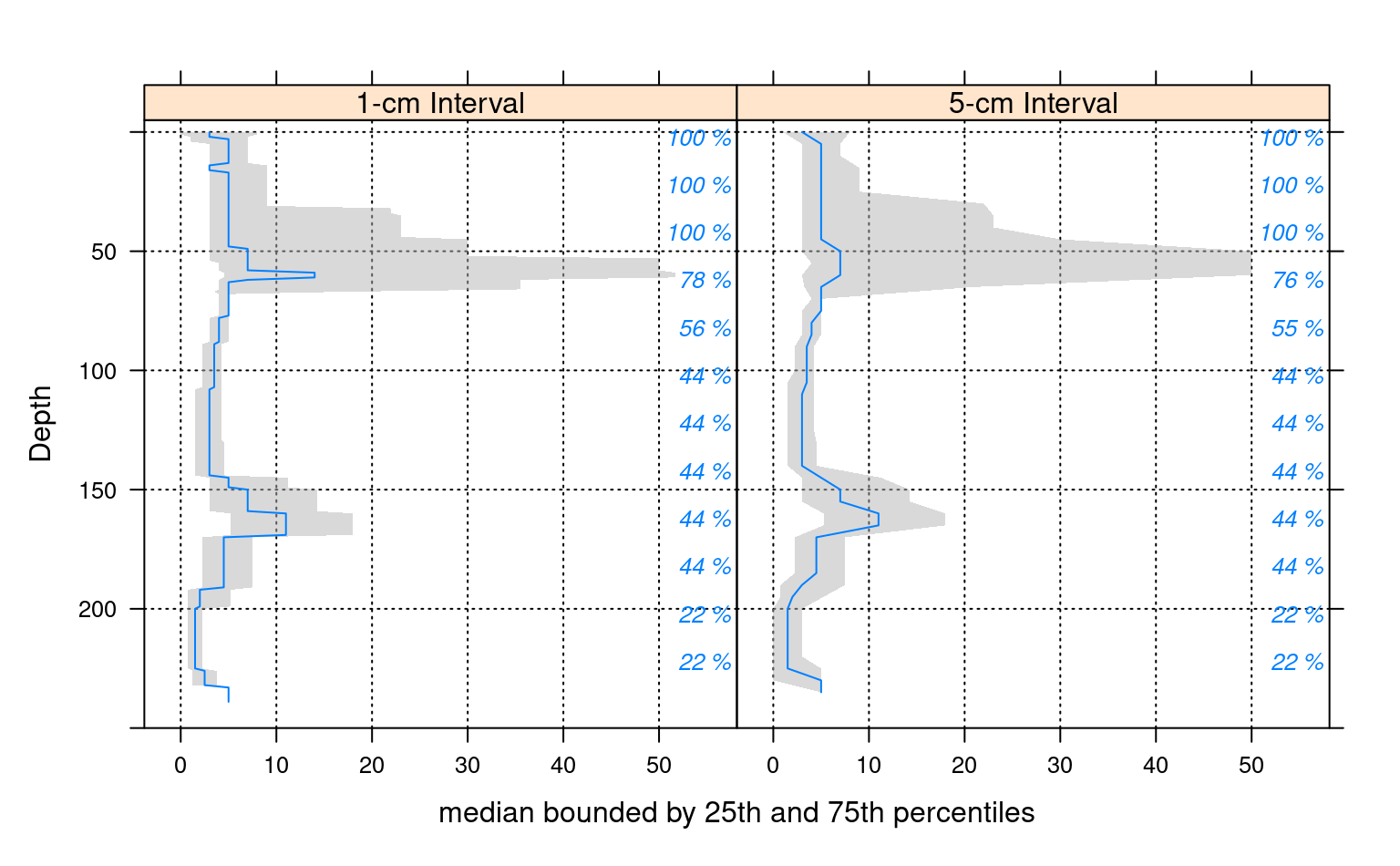### ### re-arrange data / no aggregation ### # load sample data, upgrade to SoilProfileCollection data(sp1) depths(sp1) <- id ~ top + bottom #> This is already a SoilProfileCollection-class object, doing nothing. # arrange data by ID a <- slab(sp1, fm = id ~ prop, slab.fun=identity) #> horizons with zero thickness have been omitted from results # convert id to a factor for plotting a$id <- factor(a$id) # check output str(a) #> 'data.frame': 2160 obs. of 6 variables: #>$ variable             : Factor w/ 1 level "prop": 1 1 1 1 1 1 1 1 1 1 ...
#>  $id : Factor w/ 9 levels "P001","P002",..: 1 1 1 1 1 1 1 1 1 1 ... #>$ value                : int  13 13 7 7 7 7 7 7 7 7 ...
#>  $contributing_fraction: num 1 1 1 1 1 1 1 1 1 1 ... #>$ top                  : int  0 1 2 3 4 5 6 7 8 9 ...
#>  $bottom : int 1 2 3 4 5 6 7 8 9 10 ... # plot via step function xyplot(top ~ value | id, data=a, ylab='Depth', ylim=c(250, -5), as.table=TRUE, panel=panel.depth_function, prepanel=prepanel.depth_function, scales=list(x=list(alternating=1)) )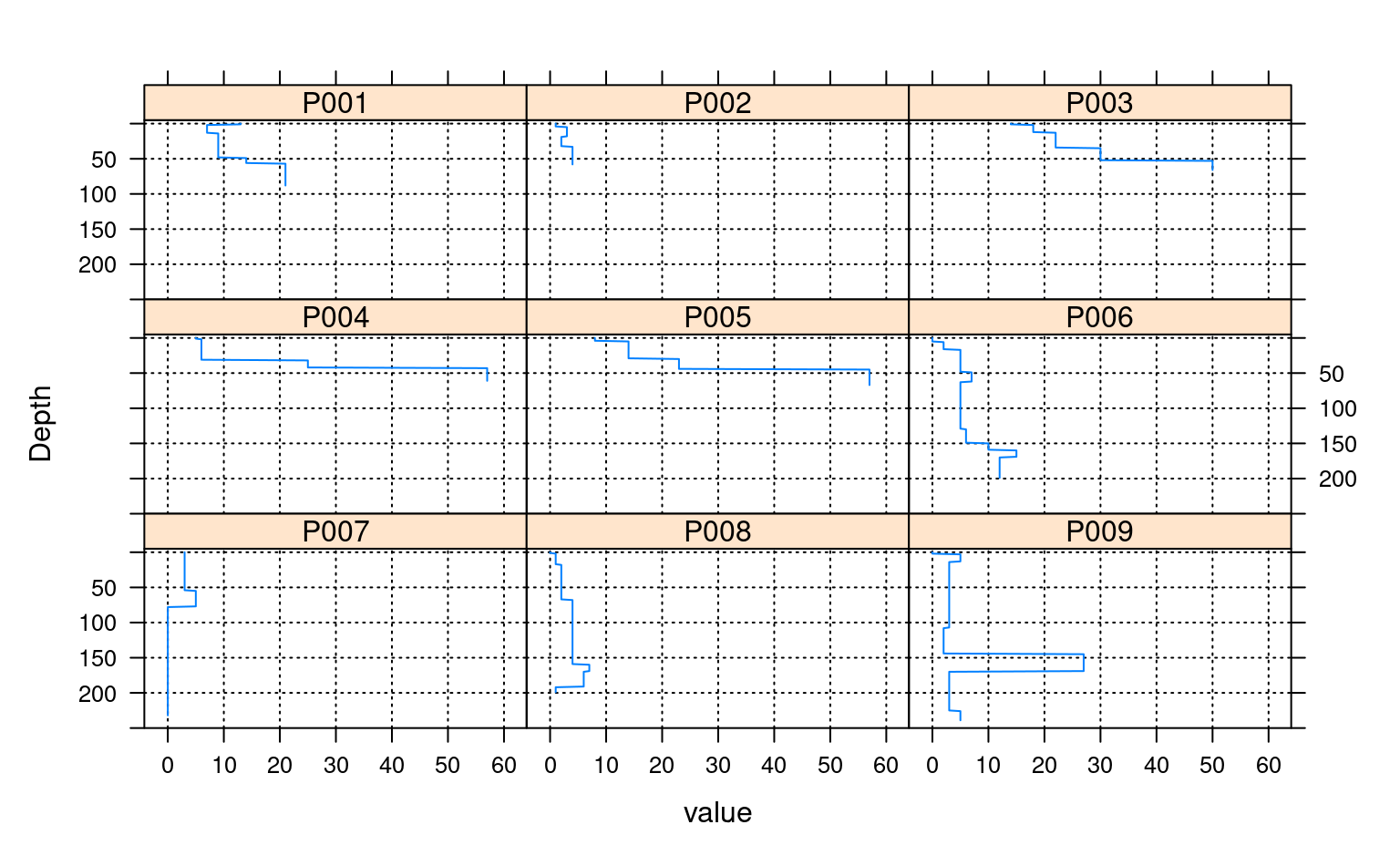## ## categorical variable example ## data(sp1) depths(sp1) <- id ~ top + bottom #> This is already a SoilProfileCollection-class object, doing nothing. # normalize horizon names: result is a factor sp1$name <- generalize.hz(
sp1$name, new = c('O','A','B','C'), pat = c('O', '^A','^B','C') ) # compute slice-wise probability so that it sums to contributing fraction, from 0-150 a <- slab(sp1, fm= ~ name, cpm=1, slab.structure=0:150) #> horizons with zero thickness have been omitted from results # convert wide -> long for plotting # result is a data.table # genhz factor levels are set by order in measure.vars a.long <- melt( as.data.table(a), id.vars = c('top','bottom'), measure.vars = c('O', 'A', 'B', 'C'), ) # plot horizon type proportions using panels xyplot(top ~ value | variable, data = a.long, subset=value > 0, col = 1, lwd = 2, xlab = 'Class Probability', ylab = 'Depth (cm)', strip = strip.custom(bg = grey(0.85)), scales = list(x = list(alternating = FALSE)), ylim = c(150, -5), type=c('S','g'), horizontal = TRUE, layout = c(4,1) )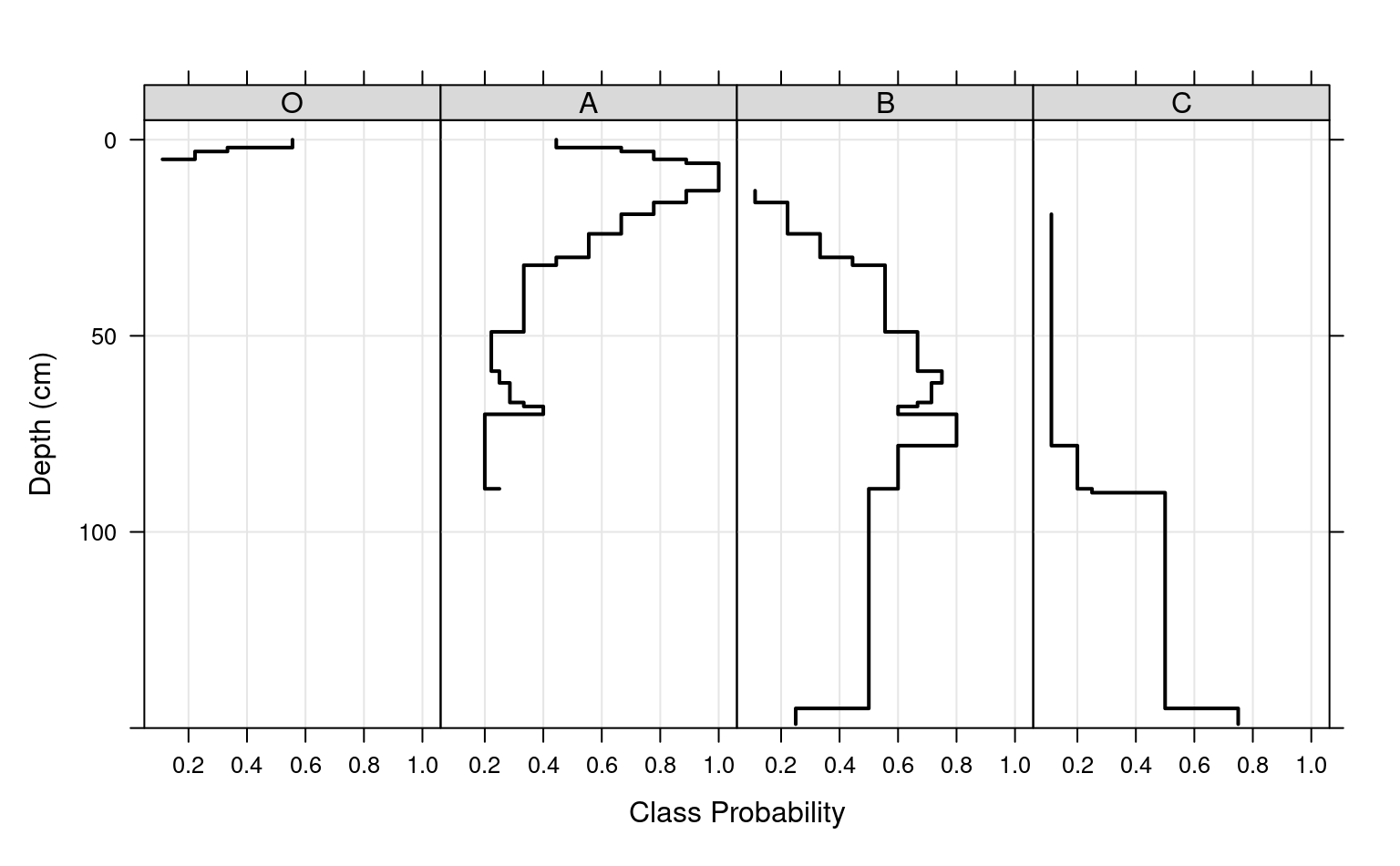# again, this time using groups xyplot(top ~ value, data = a.long, groups = variable, subset = value > 0, ylim = c(150, -5), type = c('S','g'), horizontal = TRUE, asp = 2, lwd = 2, auto.key = list( lines = TRUE, points = FALSE, cex = 0.8, columns = 1, space = 'right' ) )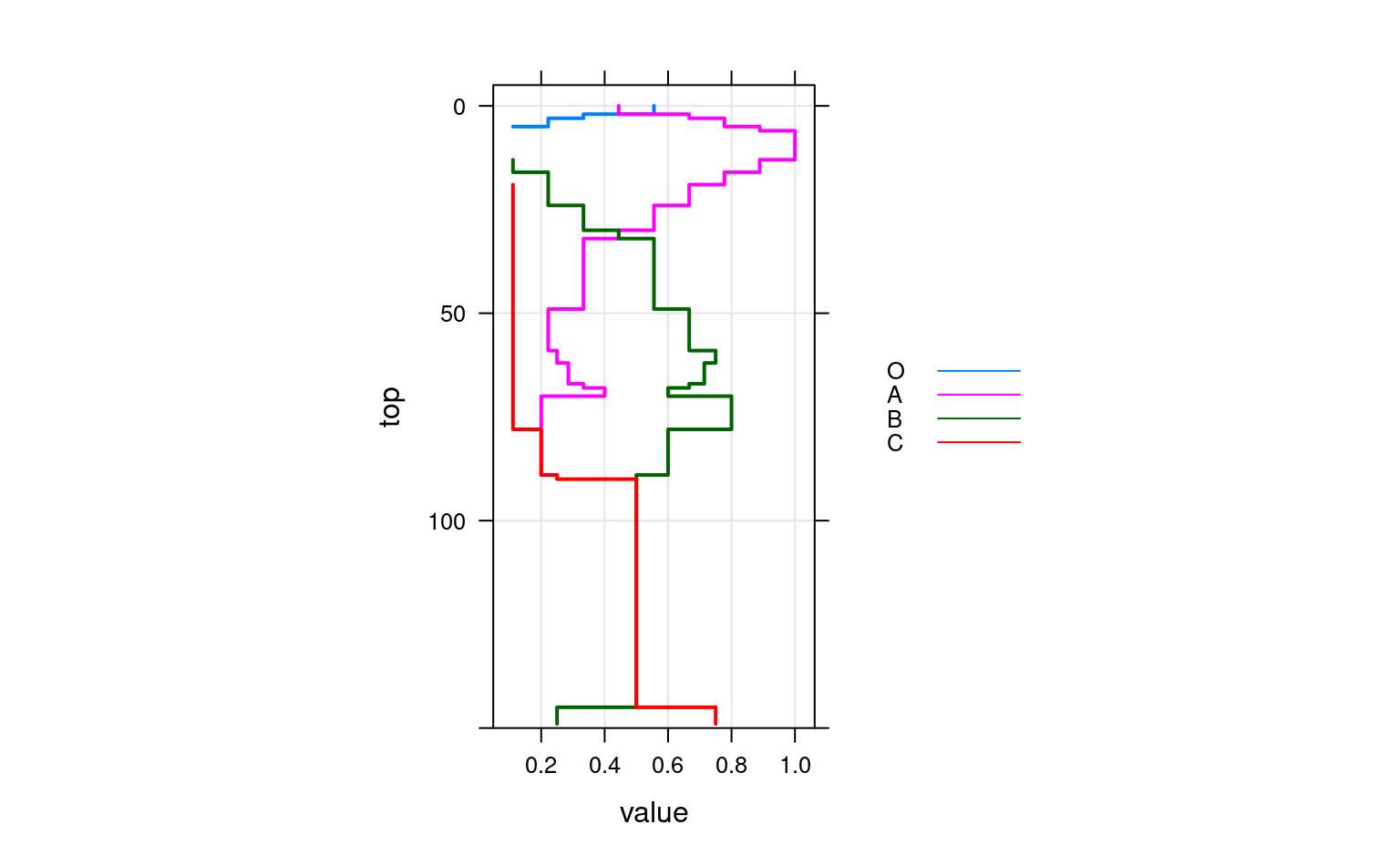# adjust probability to size of collection, from 0-150 a.1 <- slab(sp1, fm= ~ name, cpm = 2, slab.structure = 0:150) #> horizons with zero thickness have been omitted from results # convert wide -> long for plotting # result is a data.table # genhz factor levels are set by order in measure.vars a.1.long <- melt( as.data.table(a.1), id.vars = c('top','bottom'), measure.vars = c('O','A','B','C') ) # combine aggregation from cpm modes 1 and 2 g <- make.groups(cmp.mode.1 = a.long, cmp.mode.2 = a.1.long) # plot horizon type proportions xyplot(top ~ value | variable, groups = which, data = g, subset = value > 0, ylim = c(240, -5), type = c('S','g'), horizontal = TRUE, layout = c(4,1), auto.key = list(lines = TRUE, points = FALSE, columns = 2), par.settings = list(superpose.line = list(col = c(1, 2), lwd = 2)), scales = list(alternating = 3), xlab = 'Class Probability', ylab = 'Depth (cm)', strip = strip.custom(bg = grey(0.85)) )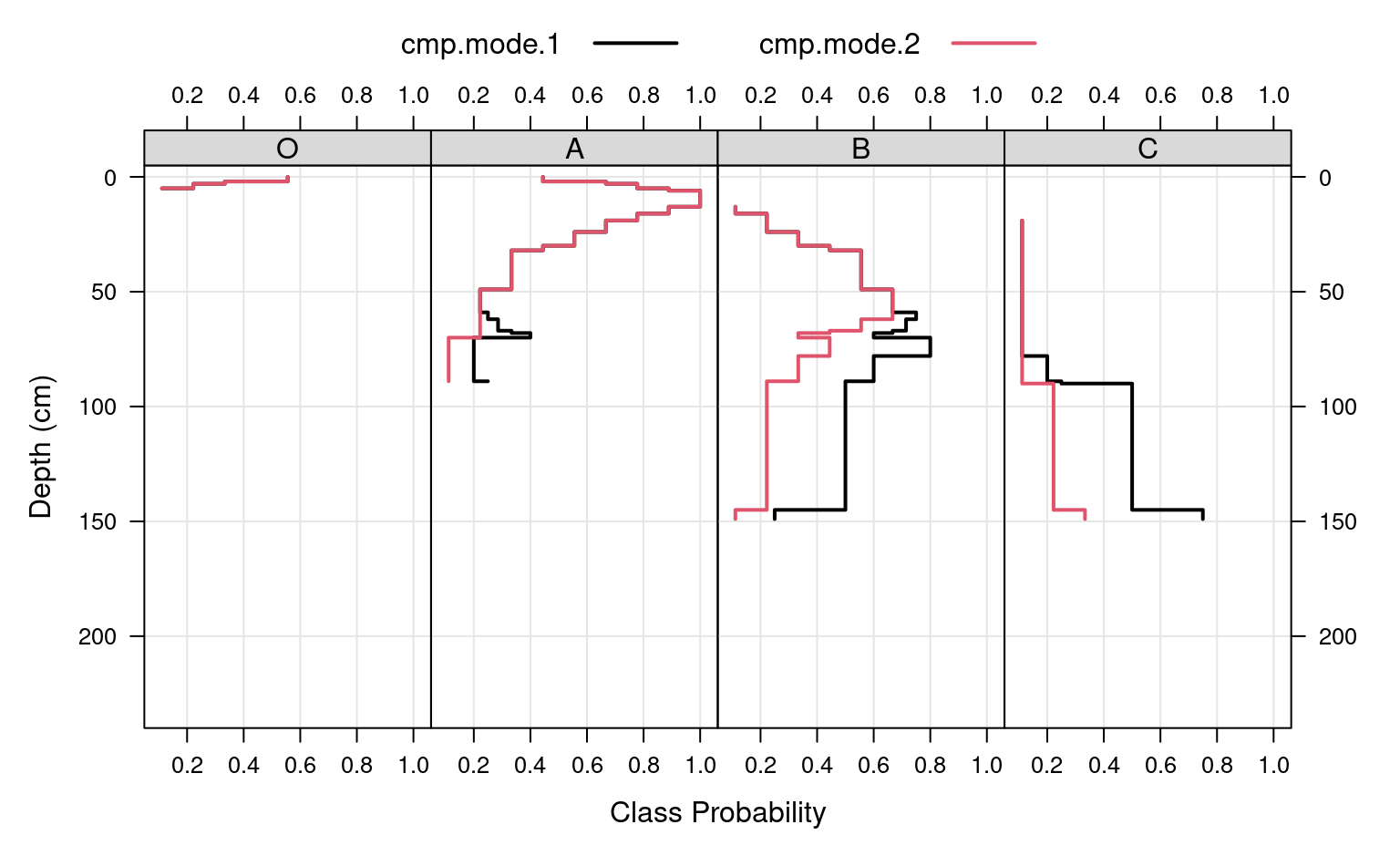# apply slice-wise evaluation of max probability, and assign ML-horizon at each slice gen.hz.ml <- get.ml.hz(a, "variable", c('O','A','B','C')) if (FALSE) { ## ## HD quantile estimator ## library(soilDB) library(lattice) library(data.table) # sample data data('loafercreek', package = 'soilDB') # defaul slab.fun wraps stats::quantile() a <- slab(loafercreek, fm = ~ total_frags_pct + clay) # use HD quantile estimator from Hmisc package instead a.HD <- slab(loafercreek, fm = ~ total_frags_pct + clay, slab.fun = aqp:::.slab.fun.numeric.HD) # combine g <- make.groups(standard=a, HD=a.HD) # note differences densityplot(~ p.q50 | variable, data=g, groups=which, scales=list(relation='free', alternating=3, tick.number=10, y=list(rot=0)), xlab='50th Percentile', pch=NA, main='Loafercreek', auto.key=list(columns=2, points=FALSE, lines=TRUE), par.settings=list(superpose.line=list(lwd=2, col=c('RoyalBlue', 'Orange2'))) ) # differences are slight but important xyplot( top ~ p.q50 | variable, data=g, groups=which, xlab='Value', ylab='Depth (cm)', asp=1.5, main='Loafercreek', lower=g$p.q25, upper=g$p.q75, sync.colors=TRUE, alpha=0.25, cf=g$contributing_fraction,
ylim=c(115,-5), layout=c(2,1), scales=list(x=list(relation='free')),
par.settings=list(superpose.line=list(lwd=2, col=c('RoyalBlue', 'Orange2'))),
strip=strip.custom(bg=grey(0.85)),
panel=panel.depth_function,
prepanel=prepanel.depth_function,
auto.key=list(columns=2, lines=TRUE, points=FALSE)
)

##
## multivariate examples
##
data(sp3)

sp3$group <- 'group 1' sp3$group[as.numeric(sp3$id) > 5] <- 'group 2' sp3$group <- factor(sp3$group) # upgrade to SPC depths(sp3) <- id ~ top + bottom site(sp3) <- ~ group # custom 'slab' function, returning mean +/- 1SD mean.and.sd <- function(values) { m <- mean(values, na.rm=TRUE) s <- sd(values, na.rm=TRUE) upper <- m + s lower <- m - s res <- c(mean=m, lower=lower, upper=upper) return(res) } # aggregate several variables at once, within 'group' a <- slab(sp3, fm = group ~ L + A + B, slab.fun = mean.and.sd) # check the results: # note that 'group' is the column containing group labels xyplot( top ~ mean | variable, data=a, groups=group, lower=a$lower, upper=a$upper, sync.colors=TRUE, alpha=0.5, cf = a$contributing_fraction,
xlab = 'Mean Bounded by +/- 1SD',
ylab = 'Depth (cm)',
ylim=c(125,-5), layout=c(3,1),
scales=list(x=list(relation='free')),
par.settings = list(superpose.line=list(lwd=2, col=c('RoyalBlue', 'Orange2'))),
panel = panel.depth_function,
prepanel = prepanel.depth_function,
strip = strip.custom(bg=grey(0.85)),
auto.key = list(columns=2, lines=TRUE, points=FALSE)
)

# compare a single profile to the group-level aggregate values
a.1 <- slab(sp3[1, ], fm = group ~ L + A + B, slab.fun = mean.and.sd)

# manually update the group column
a.1$group <- 'profile 1' # combine into a single data.frame: g <- rbind(a, a.1) # plot with customized line styles xyplot( top ~ mean | variable, data=g, groups=group, subscripts=TRUE, lower=a$lower, upper=a$upper, ylim=c(125,-5), layout=c(3,1), scales=list(x=list(relation='free')), xlab = 'Mean Bounded by +/- 1SD', ylab = 'Depth (cm)', panel=panel.depth_function, prepanel=prepanel.depth_function, sync.colors = TRUE, alpha = 0.25, par.settings = list( superpose.line = list( col = c('orange', 'royalblue', 'black'), lwd = 2, lty = c(1,1,2) ) ), strip = strip.custom(bg=grey(0.85)), auto.key = list(columns=3, lines=TRUE, points=FALSE) ) ## again, this time for a user-defined slab from 40-60 cm a <- slab(sp3, fm = group ~ L + A + B, slab.structure = c(40,60), slab.fun = mean.and.sd ) # now we have weighted average properties (within the defined slab) # for each variable, and each group # convert long -> wide dcast( as.data.table(a), formula = group + top + bottom ~ variable, value.var = 'mean' ) ## this time, compute the weighted mean of selected properties, by profile ID a <- slab(sp3, fm = id ~ L + A + B, slab.structure = c(40,60), slab.fun = mean.and.sd ) # convert long -> wide dcast( as.data.table(a), formula = id + top + bottom ~ variable, value.var = 'mean' ) ## aggregate the entire collection, using default slab function (hdquantile) ## note the missing left-hand side of the formula a <- slab(sp3, fm= ~ L + A + B) ## weighted-aggregation -- NOT YET IMPLEMENTED -- # load sample data, upgrade to SoilProfileCollection data(sp1) depths(sp1) <- id ~ top + bottom # generate pretend weights as site-level attribute set.seed(10101) sp1$site.wts <- runif(n=length(sp1), min=20, max=100)
}

`